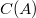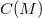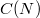﻿

### Multiplicatively closed bases for$C (A)$

#### Abstract

A canonical basis for the centralizer$C(M)$ of a$n n$ complex matrix M is obtained from the one$C(A)$ constructed for a Jordan canonical form A of M. These bases are closed under nonzero products and we obtain simple multiplication tables for their products. Two matrices have the same centralizer invariants iff their Jordan canonical forms have the same block structure. For square matrices M and N we prove that$C(M)$ and$C(N)$ are isomorphic as algebras over C iff they have the same size and the same centralizer invariants. We produce a similar canonical basis for the centralizer algebra of a real matrix over the real numbers which has analogous multiplicative properties in addition to conjugation properties. We also give some additional consequences of these results.

DOI Code: 10.1285/i15900932v26n2p81

Keywords: Centralizer algebra; Matrix; Basis; Center

Classification: 15A21; 15A30

Full Text: PDF